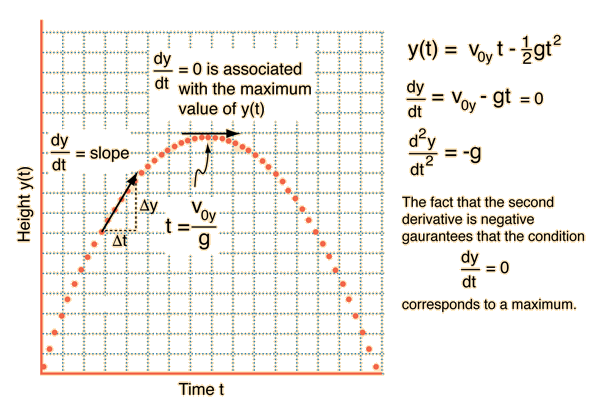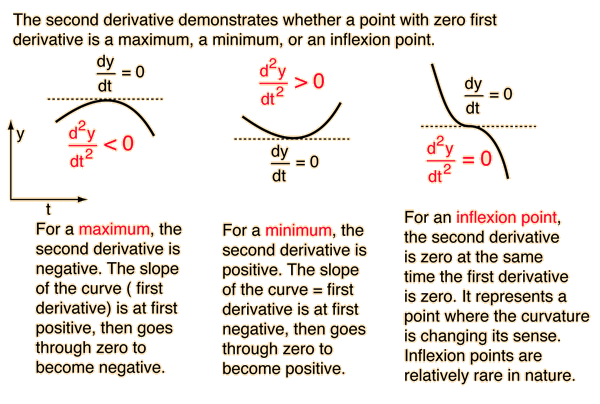We are here with you hands in hands to facilitate your learning & don't appreciate the idea of copying or replicating solutions. Read More>>

#www.vustudents.ning.com

 www.bit.ly/vucodes + Link For Assignments, GDBs & Online Quizzes Solution www.bit.ly/papersvu + Link For Past Papers, Solved MCQs, Short Notes & More

Dear Students! Share your Assignments / GDBs / Quizzes files as you receive in your LMS, So it can be discussed/solved timely. Add Discussion

 Dated: Jul 09, 13 Announcement for GDB Topic:   Discuss the applications of "Maxima and Minima Value of Functions" in various fields of life. (Be precise) Opening Date: July 15, 2013 at 12:01 AM Closing Date: July 16, 2013 at 11:59 PM Instructions: (1) Post your comments only on the concern Graded MDB forum and not on regular MDB forum. (2) Write your comments in the plain text and avoid math type symbols and figures/graphs/images as these will not appear on the board. (3) Zero marks will be given to irrelevant comments or to those which are copied from website or any other source. (5) Do not enter your comments more than once. (6) Due date will not be extended. (7) No description will be accepted through e-mail.

+ How to Join Subject Study Groups & Get Helping Material?

+ How to become Top Reputation, Angels, Intellectual, Featured Members & Moderators?

+ VU Students Reserves The Right to Delete Your Profile, If?

Views: 624

.

+ http://bit.ly/vucodes (Link for Assignments, GDBs & Online Quizzes Solution)

+ http://bit.ly/papersvu (Link for Past Papers, Solved MCQs, Short Notes & More)

### Replies to This Discussion

Our main purpose here discussion not just Solution

We are here with you hands in hands to facilitate your learning and do not appreciate the idea of copying or replicating solutions.

Local Maxima

The y-value f(c) is a local maximum value (also called relative maximum value) of f if there is an open interval containing the x-value c. When the graph of the function f, continuous at x=c, is increasing on the immediate left of the number x=c and decreasing on the immediate right of the number x=c, then the value of f at c is locally the largest, i.e., f(c) is a local maximum. This test can be extended to endpoints in the domain of f: if x=c is a left endpoint and decreasing on the immediate right, or if x=c is a right endpoint and increasing on the immediate left, then f(c) is a local maximum.
Local Minima

The y-value f(c) is a local minimum value (also called relative minimum value) of f if there is an open interval containing the x-value c. When the graph of the function f, continuous at x=c, is decreasing on the immediate left of the number x=c and increasing on the immediate right of the number x=c, then the value of f at c is locally the smallest, i.e., f(c) is a local minimum. This test can be extended to endpoints in the domain of f: if x=c is a left endpoint and increasing on the immediate right, or if x=c is a right endpoint and decreasing on the immediate left, then f(c) is a local minimum.

Determining Local Maxima and Local Minima

Any value of x in the domain of f is called a critical number of f' (also called critical point or critical value) if either f'(x)=0 or f'(x) does not exist. For continuous functions, the local maxima and local minima can only occur at the critical numbers or endpoints of the domain of f. These numbers separate the domain of f into intervals. At each critical number or endpoint, there are three possibilities.
If the sign of f'(c) is positive on the left side (interval to the left) of a critical number of f' and negative on the right side, suggesting visually that the function is increasing on the left side and decreasing on the right side, then f has a local maximum at that critical number. If the sign of f'(c) is positive on the left side of a right endpoint or negative on the right side of a left endpoint, then f has a local maximum at that endpoint.
If the sign of f'(c) is negative on the left side of a critical number of f' and positive on the right side, suggesting visually that the function is decreasing on the left side and increasing on the right side, then f has a local minimum at that critical number. If the sign of f'(c) is negative on the left side of a right endpoint or negative on the right side of a left endpoint, then f has a local minimum at that endpoint.
If there is no change in sign of f'(c) from either side of a critical number to the other side, then the critical number is not a local maximum or local minimum for f. The curve has a horizontal tangent at the critical number but the point of tangency is not a turning point.

Function can have “hills and valleys” places where they reach a maximum and minimum values. It may not be the minimum or maximum for the whole function. I our daily life we saw many hills and valleys. The hills are maximum point and the valleys are the minimum point. The plural of minimum is Minima and the plural of maximum is Maxima. Maxima and Minima are collectively called Extrema.The process of a finding maximum or minimum values is called optimization. Maximum or minimum can be defined as largest and smallest of function at a given point in it Domain or outside its domain. Local maxima is the Point that is at the peak with respect to the local surrounding points. Similarly, local minima can be defined as the lowest point with respect to the surrounding points. Maxima or minima can be many points as they are considered locally in the graph. It also resembles with the Set theory in a context that we have least and peak points in the set. In our life the Government or an industry regulatory can set a maximum price in an attempt to prevent the market price from rising above a certain level . The minimum price is a price below which the market price cannot fall.

, any applications of maxima and minima are purely graphical:

• , we have used the absolute maxima of wavefunctions and radial probability distribution functions to determine where an electron is most likely to be found in any given orbital.

• In PHYS 101, the absolute maximum (or minimum) displacement of a wave is known as its amplitude, and is occasionally found graphically. We have also solved equations to determine the maxima of velocity and acceleration functions for waves, using other physical principles (such as the Law of Conservation of Energy).

If you are taking ECON 101 as an elective course this term, you are likely familiar with the many applications of maxima and minima in the world of economics. Maxima and minima are used to maximize beneficial values (profit, efficiency, output, etc.) and to minimize negative ones (expenses, effort, etc.).

Applications: "Real World"

Maxima and minima pop up all over the place in our daily lives. They can be found anywhere we are interested in the highest and/or lowest value of a given system; if you look hard enough, you can probably find them just about anywhere! Here are just a few examples of where you might encounter maxima and minima:

• A meteorologist creates a model that predicts temperature variance with respect to time. The absolute maximum and minimum of this function over any 24-hour period are the forecasted high and low temperatures, as later reported on The Weather Channel or the evening news.

• The director of a theme park works with a model of total revenue as a function of admission price. The location of the absolute maximum of this function represents the ideal admission price (i.e., the one that will generate the most revenue).

• An actuary works with functions that represent the probability of various negative events occuring. The local minima of these functions correspond to lucrative markets for his/her insurance company – low-risk, high-reward ventures.

• A NASA engineer working on the next generation space shuttle studies a function that computes the pressure acting on the shuttle at a given altitude. The absolute maximum of this function represents the pressure that the shuttle must be designed to sustain.

• A conscientious consumer meticulously collects data on his/her cell phone usage over a certain interval (say, a month) and develops a function to represent cell phone usage over time. The local maxima and minima of this function give the consumer a better idea of his/her usage patterns terns, empowering him/her to choose the most appropriate cell phone plan.

MIT Student gud keep it up & thanks for sharing

# Maxima and Minima from Calculus

One of the great powers of calculus is in the determination of the maximum or minimum value of a function. Take f(x) to be a function of x. Then the value of x for which the derivative of f(x) with respect to x is equal to zero corresponds to a maximum, a minimum or an inflexion point of the function f(x).

For example, the height of a projectile that is fired straight up is given by the motion equation:

Taking y0 = 0, a graph of the height y(t) is shown below.The derivative of a function can be geometrically interpreted as the slope of the curve of the mathematical function y(t) plotted as a function of t. The derivative is positive when a function is increasing toward a maximum, zero (horizontal) at the maximum, and negative just after the maximum. The second derivative is the rate of change of the derivative, and it is negative for the process described above since the first derivative (slope) is always getting smaller. The second derivative is always negative for a "hump" in the function, corresponding to a maximum.